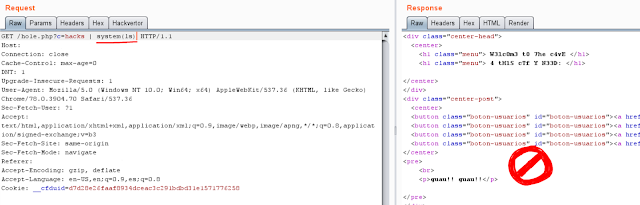# XORpass - An encoder to bypass WAFXORpass is an encoder to bypass WAF filters using XOR operations.

Installation & Usage

``git clone https://github.com/devploit/XORpasscd XORpass\$ python3 xorpass.py -h``
Example of bypass:
Using clear PHP function:Using XOR bypass of that function:
``\$ python3 xorpass.py -e "system(ls)"``### Why does PHP treat our payload as a string?

The ^ is the exclusive or operator, which means that we're in reality working with binary values. So let's break down what happens.

The XOR operator on binary values will return 1 where just one of the bits were 1, otherwise it returns 0 (0^0 = 0, 0^1 = 1, 1^0 = 1, 1^1 = 0). When you use XOR on characters, you're using their ASCII values. These ASCII values are integers, so we need to convert those to binary to see what's actually going on.
``A = 65 = 1000001S = 83 = 1010011B = 66 = 1000010A       1000001        ^S       1010011        ^B       1000010----------------result  1010000 = 80 = PA^S^B = P``

If we do an 'echo "A"^"S"^"B";' PHP will return us a P as we see.